# Exponent Worksheets

Exponent worksheets contain identifying base and index, writing exponents in standard and exponents form, evaluation expression involving exponents, simplifying expression by applying the laws of exponents and more.

## Basic Exponent Worksheets

Identify the Base and Exponents:

Identify the base and index (exponent) in each problem. In pre-algebra, bases are numerals whereas in Algebra, bases are variables.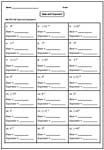Pre-Algebra:

Algebra:

Expanded Form and Exponent Form:

Write each problem in expanded form or exponent form.Easy:

Moderate:

Difficult:

Mixed Review: Includes all 3 Forms

This section help kids to practice standard, expanded and exponent form in each worksheet.Easy:

Moderate:

Difficult:

Old Worksheets:

Exponent Form

## Intermediate Exponent Worksheets

Evaluating Expression

Find the value of the exponents in each term and then solve. Each worksheet includes all the four major operations; addition, subtraction, multiplication and division.Easy:

Moderate:

Difficult:

Find the Missing Base or Exponent

An exponential equation contains the variable in either base or exponent. Identify the missing base or exponent to balance the equation.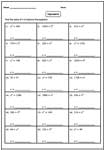Easy:

Moderate:

Difficult:

Old Worksheets:

## Laws of Exponents or Indices

Product Rule Worksheets

Use product rule to simplify each expression and write the answers in positive exponents.Pre-Algebra:

Algebra:

Easy:

Moderate:

Difficult:

Quotient Rule Worksheets

Apply quotient rule to simplify the expressions. Pre-algebra section includes 21 questions and Algebra includes 18 questions in each worksheet.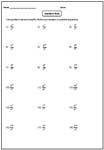Pre-Algebra:

Algebra:

Easy:

Moderate:

Difficult:

Power Rule Worksheets

Apply power rule to express each problem in single exponent.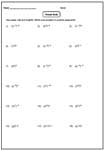Pre-Algebra:

Algebra:

Easy:

Moderate:

Difficult:

Laws of Exponents (indices): Mixed Review

Apply the laws of exponents that are necessary to simplify the expression. This section is good to review all the laws in exponents.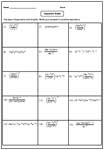Pre-Algebra:

Easy:

Moderate:

Difficult:

Algebra:

Easy:

Moderate:

Difficult:

Old Worksheets:

Product Rule:

Quotient Rule:

Power Rule:

All Rules:

Stock up your library with the complete set of worksheets on exponents.

Related Worksheets